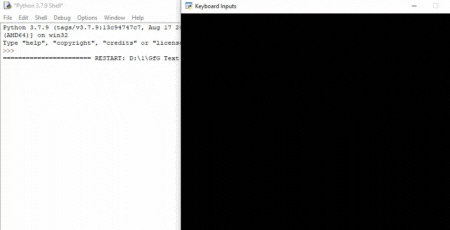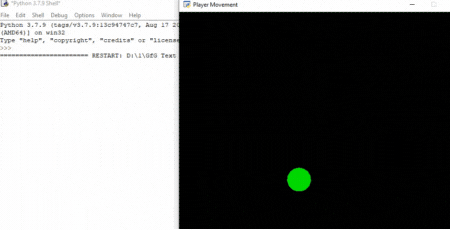Related Articles

# Python Arcade – Handling Keyboard Input

• Difficulty Level : Hard
• Last Updated : 23 Sep, 2021

In Arcade, you can easily check which keyboard button is pressed and perform tasks according to that.

For this, we are going to use these functions:

• on_key_press()
• on_key_release()

Syntax:

• on_key_press(symbol,modifiers)
• on_key_release (symbol, modifiers)

Parameters:

• symbol: Key that was hit
• modifiers: Bitwise ‘and’ of all modifiers (shift, ctrl, num lock) pressed during this event.

on_key_press() function will be called whenever the user presses a keyboard button. Similarly, on_key_released() will be called whenever a user releases a keyboard button.

## Example 1:

In this example, we will create a simple program using the arcade module which will check if the upper arrow key is pressed or not.

Then we will create three functions inside this class.

• on_draw(): Inside this function, we will start the rendering using arcade.start_render().
• on_key_press(): This function will be called whenever a keyboard key is pressed. Inside this function, we will check if the key pressed is the up arrow key then we will print “upper arrow key is pressed”.
• on_key_release(): This function will be called whenever a keyboard key is released. Inside this function, we will check if the key released is the up arrow key then we will print “upper arrow key is released”.

Below is the implementation:

## Python3

 `# Importing arcade module``import` `arcade`` ` `# Creating MainGame class       ``class` `MainGame(arcade.Window):``    ``def` `__init__(``self``):``        ``super``().__init__(``600``, ``600``, title``=``"Keyboard Inputs"``)`` ` `    ``# Creating on_draw() function to draw on the screen``    ``def` `on_draw(``self``):``        ``arcade.start_render()``         ` `    ``# Creating function to check button is pressed``    ``# or not``    ``def` `on_key_press(``self``, symbol,modifier):`` ` `        ``# Checking the button pressed``        ``# is up arrow key or not``        ``if` `symbol ``=``=` `arcade.key.UP:``            ``print``(``"Upper arrow key is pressed"``)`` ` `    ``# Creating function to check button is released``    ``# or not``    ``def` `on_key_release(``self``, symbol, modifier):`` ` `        ``# Checking the button pressed``        ``# is up arrow key or not``        ``if` `symbol ``=``=` `arcade.key.UP:``            ``print``(``"Upper arrow key is released"``)``         ` `# Calling MainGame class       ``MainGame()``arcade.run()`

Output:## Example 2:

In this example, we move the player according to the keyboard inputs.

For this, we are going to create a MainGame() class. Inside this class first, we are going to initialize some variables for x and y coordinates of the player’s sprite and player’s x and y velocity then we will create 4 functions inside this class.

• on_draw(): Inside this function, we will draw our player and start the rendering.
• setup(): In this function, we will initialize our camera and scene object then we will load our player and platform’s sprites. After that, we will call the  PhysicsEnginePlatformer() function.
• on_update(): In this function, we will update the x and y coordinates of the player’s sprite by adding the value of vel_x and vel_y variable,
• on_key_press(): In this function, we will change the value of the vel_x and vel_y variables according to the keyboard key that is pressed.
• on_key_release(): In this function, we will change the value of the vel_x and vel_y variables according to the keyboard key that is released.

## Python3

 `# Importing arcade module``import` `arcade`` ` `# Creating MainGame class       ``class` `MainGame(arcade.Window):``    ``def` `__init__(``self``):``        ``super``().__init__(``600``, ``600``, title``=``"Player Movement"``)`` ` `        ``# Initializing the initial x and y coordinated``        ``self``.x ``=` `250` `        ``self``.y ``=` `250`` ` `        ``# Initializing a variable to store``        ``# the velocity of the player``        ``self``.vel_x ``=` `0``        ``self``.vel_y ``=` `0`` ` `    ``# Creating on_draw() function to draw on the screen``    ``def` `on_draw(``self``):``        ``arcade.start_render()`` ` `        ``# Drawing the rectangle using``        ``# draw_rectangle_filled function``        ``arcade.draw_circle_filled(``self``.x, ``self``.y,``25``,``                                     ``arcade.color.GREEN )``    ``# Creating on_update function to``    ``# update the x coordinate``    ``def` `on_update(``self``,delta_time):``        ``self``.x ``+``=` `self``.vel_x ``*` `delta_time``        ``self``.y ``+``=` `self``.vel_y ``*` `delta_time`` ` `         ` `    ``# Creating function to change the velocity``    ``# when button is pressed``    ``def` `on_key_press(``self``, symbol,modifier):`` ` `        ``# Checking the button pressed``        ``# and changing the value of velocity``        ``if` `symbol ``=``=` `arcade.key.UP:``            ``self``.vel_y ``=` `300``            ``print``(``"Up arrow key is pressed"``)``        ``elif` `symbol ``=``=` `arcade.key.DOWN:``            ``self``.vel_y ``=` `-``300``            ``print``(``"Down arrow key is pressed"``)``        ``elif` `symbol ``=``=` `arcade.key.LEFT:``            ``self``.vel_x ``=` `-``300``            ``print``(``"Left arrow key is pressed"``)``        ``elif` `symbol ``=``=` `arcade.key.RIGHT:``            ``self``.vel_x ``=` `300``            ``print``(``"Right arrow key is pressed"``)`` ` `    ``# Creating function to change the velocity``    ``# when button is released``    ``def` `on_key_release(``self``, symbol, modifier):`` ` `        ``# Checking the button released``        ``# and changing the value of velocity``        ``if` `symbol ``=``=` `arcade.key.UP:``            ``self``.vel_y ``=` `0``        ``elif` `symbol ``=``=` `arcade.key.DOWN:``            ``self``.vel_y ``=` `0``        ``elif` `symbol ``=``=` `arcade.key.LEFT:``            ``self``.vel_x ``=` `0``        ``elif` `symbol ``=``=` `arcade.key.RIGHT:``            ``self``.vel_x ``=` `0``         ` `# Calling MainGame class       ``MainGame()``arcade.run()`

Output:Attention geek! Strengthen your foundations with the Python Programming Foundation Course and learn the basics.

To begin with, your interview preparations Enhance your Data Structures concepts with the Python DS Course. And to begin with your Machine Learning Journey, join the Machine Learning – Basic Level Course

My Personal Notes arrow_drop_up